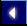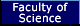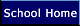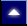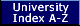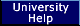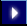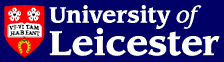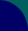# School of Mathematics & Computer Science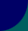# Technical Reports 2002

## Summary

2002/01 D. Kuske and M. Lohrey.

On the theory of one-step rewriting in trace monoids

2002/02 Duncan W. Parkes and Richard M. Thomas.

Groups with context-free reduced word problem

2002/03 A. Henke and A. Regev.

Weyl Modules for the Schur Algebra of the Alternating Group

2002/04 P. Houston, I. Perugia and D. Schotzau.

hp-DGFEM for Maxwell's equations

2002/05 R. Hartmann and P. Houston.

Goal-Oriented A Posteriori Error Estimation for Compressible Fluid Flows

2002/06 P. Houston, B. Senior and E. Suli.

Sobolev Regularity Estimation for hp-Adaptive Finite Element Methods

2002/07 R. Hartmann and P. Houston.

Adaptive Discontinuous Galerkin Finite Element Methods for the Compressible Euler Equations.

2002/08 E. Suli and P. Houston.

Adaptive Finite Element Approximation of Hyperbolic Problems.

2002/09 Yifeng Chen and Zhiming Liu.

Unifying Temporal Logics

2002/10 A. Baranov.

Finitary Lie algebras

2002/11 Irek Ulidowski and Shoji Yuen.

Process Languages for Rooted Weak Preorders

2002/12 A. Henke and A. Regev.

A-Codimensions and A-Cocharacters

2002/13 I. A. Stewart.

The complexity of achievement and maintenance problems in agent-based systems

2002/14 Heinz Langer, Matthias Langer and Christiane Tretter.

Variational principles for eigenvalues of block operator matrices

2002/15 Catharina Stroppel.

Category O : Gradings and Translation Functors

2002/16 Rajeev Raman, Venkatesh Raman and S. Srinivasa Rao.

Succinct Indexable Dictionaries with Applications to Encoding $k$-ary Trees, Prefix Sums and Multisets

2002/17 Jeremy Levesley.

Multilevel Scattered Data Approximation by Adaptive Domain Decomposition

2002/18 Jeremy Levesley.

Estimates of n-Widths of Sobolev's Classes on Compact Globally Symmetric Spaces of Rank 1

2002/20 Nobuko Yoshida.

Type-Based Liveness Guarantee in the Presence of Nontermination and Nondeterminism

2002/21 Irek Ulidowski.

Termination and confluence of term rewrite systems for GSOS process languages

2002/22 Jose Luis Vivas and Nobuko Yoshida.

Dynamic Channel Screening in the Higher Order Pi-Calculus

2002/23 Matthias Langer and David Eschwe.

Variational principles for eigenvalues of self-adjoint pencils of unbounded operators

2002/24 Zhiming Liu, Xiaoshan Li and Jifeng He.

Using Transition Systems to Unify UML Requirement Models

2002/25 Torben Hagerup and Rajeev Raman.

An Efficient Quasidictionary

2002/26 Danny Krizanc, Flaminia L. Luccio and Rajeev Raman.

Compact Routing Schemes for Dynamic Ring Networks

2002/27 Paula Severi and Femke van Raamsdonk.

Eliminating proofs from programs

2002/28 Paula Severi and Fer-Jan de Vries.

An Extensional Bohm Model

2002/29 Paula Severi and Fer-Jan de Vries.

A lambda calculus for D-infinity

2002/30 Irek Ulidowski.

Priority rewrite systems for OSOS process languages

2002/31 Robert Marsh, Markus Reineke and Andrei Zelevinsky.

Generalized associahedra via quiver representations

2002/32 Ralf Hartmann and Paul Houston.

Goal-Oriented A Posteriori Error Estimation for Multiple Target Functionals

2002/33 Kathryn Harriman, Paul Houston, Bill Senior and Endre Suli.

hp-Version Discontinuous Galerkin Methods with Interior Penalty for Partial Differential Equations with Nonnegative Characteristic Form

2002/34 A. K. Kushpel, J. Levesley and S. Tozoni.

Estimates of $n$--Widths of Besov Classes on Two-Point Homogeneous Manifolds

2002/35 Rachel Taillefer.

Injective Hopf bimodules, cohomologies of infinite dimensional Hopf algebras and graded-commutativity of the Yoneda product

2002/36 A. Henke and S. Doty.

Decomposition of tensor products of modular irreducibles for SL_2

2002/37 Dietrich Kuske and Markus Lohrey.

Decidable Theories of Graphs, Factorized Unfoldings and Cayley-graphs

2002/38 Manfred Droste and Dietrich Kuske.

Skew and infinitary formal power series

2002/39 Dietrich Notbohm and Nitu Kitchloo.

Quasi finite loop spaces are manifolds

2002/40 Dietrich Notbohm.

2-compact groups of rank 2

2002/41 R. Marsh and M. Reineke.

Canonical basis linearity regions arising from quiver representations

2002/42 Steve Lakin.

Context-sensitive decision problems in groups (PhD thesis)

2002/43 Steffen Koenig, Katsunori Sanada and Nicole Snashall.

On Hochschild Cohomology of Orders

2002/44 J. Levesley, C. Odell and D. L. Ragozin.

Variational Interpolation on Homogeneous Manifolds: the Norming Set Approach

2002/45 Paul Houston, Ilaria Perugia and Dominik Schotzau.

Mixed discontinuous Galerkin approximation of the Maxwell operator

2002/46 E.L.Green, Nicole Snashall and R.Taillefer.

Drinfel'd Double of Taft Algebras

2002/47 A. Momigliano and S. J. Ambler.

Multi-Level Meta-Reasoning with Higher-Order Abstract Syntax

2002/48 Nicole Snashall and Oeyvind Solberg.

Support Varieties and Hochschild Cohomology Rings

Coordination Technologies for Just-In-Time Integration

2002/50 Antonia Lopes and Jose Luiz Fiadeiro.

Superposition: Composition vs Refinement of Non-deterministic, Action-Based Systems

Service-Oriented Business and System Specification : Beyond Object-Orientation

## Full details

2002/01 D. Kuske and M. Lohrey.

On the theory of one-step rewriting in trace monoids

We introduce a general class of rewriting systems over trace monoids, called scattered rewriting systems, which generalize trace rewriting systems. We prove that the first-order theory of the one-step rewriting relation associated with a scattered rewriting system is decidable but in general not elementary. This extends known results on semi-Thue systems but our proofs use new methods; these new methods yield the decidability of local properties expressed in first-order logic augmented by modulo-counting quantifiers. Using the main decidability result, we define several subclasses of trace rewriting systems for which the confluence problem is decidable.

Available as: gzipped PostScript (.ps.gz)

2002/02 Duncan W. Parkes and Richard M. Thomas.

Groups with context-free reduced word problem

In this report we consider reduced word problems of groups. We explain the relationship between the word problem and the reduced word problem, and we give necessary and sufficient conditions for a language to be the word problem (or the reduced word problem) of a group. In addition, we show that the reduced word problem is recursive (or recursively enumerable) precisely when the word problem is recursive. We then prove that the groups which have context-free reduced word problem with respect to some finite momoid generating set are exactly the context-free groups, thus proving a conjecture of Haring-Smith. We also show that, if a group G has finite irreducible word problem with respect to a monoid generating set X, then the reduced word problem of G with respect to X is simple; this is a generalization of one direction of a theorem of Haring-Smith.

2002/03 A. Henke and A. Regev.

Weyl Modules for the Schur Algebra of the Alternating Group

Let $F$ be the field of complex numbers and $V=V_0 \oplus V_1$ a vector space over $F$ with $\dim V_0 =\dim V_1$. The symmetric group acts on $V^{\otimes n}$ by the sign-permutation action. Let $S^*(V,n) \subset S^*_A(V,n)$ be the corresponding Schur algebras of $S_n$ and of $A_n \subset S_n$ respectively where $A_n$ is the alternating group. Following the fundamental work of H.~Weyl, the explicit decomposition of $V^{\otimes n}$ as an $S^*(V,n)$-module was given in work by Berele and Regev. By appylying the character theory and the representation theory of $A_n$ we give here the explicit decomposition of $V^{\otimes n}$ as an $S^*_A(V,n)$-module.

2002/04 P. Houston, I. Perugia and D. Schotzau.

hp-DGFEM for Maxwell's equations

We propose hp-version interior penalty discontinuous Galerkin methods for the discretization of the curl-curl operator with divergence free constraint, often encountered in electromagnetic problems. For unstructured meshes with hanging nodes, we present error estimates that are optimal in the meshsize h and slightly suboptimal in the polynomial approximation order p. The performance of these methods is numerically tested for two-dimensional model problems.

Available as: gzipped PostScript (.ps.gz)

2002/05 R. Hartmann and P. Houston.

Goal-Oriented A Posteriori Error Estimation for Compressible Fluid Flows

We consider so-called goal-oriented' a posteriori error estimation for discontinuous Galerkin finite element approximations to the compressible Euler equations of gas dynamics. By employing a hyperbolic duality argument, we derive weighted, or Type I, a posteriori error estimates which bound the error measured in terms of certain target functionals of real or physical interest. The practical advantages of this general approach are illustrated by a series of numerical experiments.

Available as: gzipped PostScript (.ps.gz)

2002/06 P. Houston, B. Senior and E. Suli.

Sobolev Regularity Estimation for hp-Adaptive Finite Element Methods

In this paper we develop an algorithm for estimating the local Sobolev regularity index of a given function by monitoring the decay rate of its Legendre expansion coefficients. On the basis of these local regularities, we design and implement an hp-adaptive finite element method based on employing discontinuous piecewise polynomials, for the approximation of nonlinear systems of hyperbolic conservation laws. The performance of the proposed adaptive strategy is demonstrated numerically.

Available as: gzipped PostScript (.ps.gz)

2002/07 R. Hartmann and P. Houston.

Adaptive Discontinuous Galerkin Finite Element Methods for the Compressible Euler Equations.

In this paper a recently developed approach for the design of adaptive discontinuous Galerkin finite element approximations is applied to physically relevant problems arising in inviscid compressible fluid flows governed by the Euler equations of gas dynamics. In particular, we employ so-called weighted or Type I a posteriori error bounds to drive adaptive finite element algorithms for the estimation of the error measured in terms of general linear and nonlinear target functionals of the solution; typical examples considered here include the point evaluation of a component of the solution vector, and the drag and lift coefficients of a body immersed in an inviscid fluid. This general approach leads to the design of economical finite element meshes specifically tailored to the computation of the target functional of interest, as well as providing reliable and efficient error estimation. Indeed, the superiority of the proposed approach over standard mesh refinement algorithms which employ ad hoc error indicators will be illustrated by a series of numerical experiments; here, we consider transonic flow through a nozzle, as well as subsonic, transonic and supersonic flows around different airfoil geometries.

Available as: gzipped PostScript (.ps.gz)

2002/08 E. Suli and P. Houston.

Adaptive Finite Element Approximation of Hyperbolic Problems.

We review some recent developments concerning the a posteriori error analysis of h- and hp-version finite element approximations to hyperbolic problems. The error bounds stem from an error representation formula which equates the error in an output functional of interest to the inner product of the finite element residual with the solution of a dual (adjoint) problem whose data is the density function of the target functional. Type I a posteriori error bounds are derived which, unlike the cruder Type II bounds, retain the dual solution in the bound as a local weight-function. The relevance of Type I a posteriori bounds is argued by showing that the local size of the error in a hyperbolic problem may be only very weakly correlated to the local size of the residual; consequently, adaptive refinement algorithms based on the size of the local residual alone can be ineffective. The sharpness of Type I a posteriori error bounds is demonstrated on both structured and adaptively refined meshes.

Available as: gzipped PostScript (.ps.gz)

2002/09 Yifeng Chen and Zhiming Liu.

Unifying Temporal Logics

In this paper, we use a technique called {\em generic composition} to unify temporal logics. Predicates are treated as sets. Temporal operators are defined as functions, and their axioms become consequent laws. The underlying semantics of this approach corresponds to Kripke's relational semantics and is closely related to logic. Calculational reasoning involving different temporal logics is supported. It turns out that the modalities of possibility and necessity become generic composition and its inverse of converse respectively. Transformers between temporal logics also become modalities. Various temporal domains are unified under a concept called {\em resource cumulation}. Generic composition provides high-level constructions and laws for the reasoning of temporal operators. Its completeness theorem guarantees that generic composition and its inverse have the same expressiveness as the first-order logic.

2002/10 A. Baranov.

Finitary Lie algebras

An algebra is called finitary if it consists of finite-rank transformations of a vector space. We classify finitary simple and finitary irreducible Lie algebras over an algebraically closed field of characteristic different from 2 and 3.

2002/11 Irek Ulidowski and Shoji Yuen.

Process Languages for Rooted Weak Preorders

We present a general class of process languages for rooted eager bisimulation preorder and prove its congruence result. Also, we present classes of process languages for the rooted versions of several other weak preorders. The process languages we propose are defined by the {\em Ordered\/} SOS method which combines the traditional SOS approach, based on transition rules, with the {\em ordering\/} on transition rules. This new feature specifies the order of application of rules when deriving transitions of process terms. We support and illustrate our results with examples of process operators and process languages which benefit from the OSOS formulation.

2002/12 A. Henke and A. Regev.

A-Codimensions and A-Cocharacters

The codimensions and the cocharacters of a p.i.algebra arise from the group algebra FS_n of the symmetric group S_n, where F is an algebraically closed field of characteristic zero. The subalgebra FA_n of the alternating subgroup A_n gives rise to the corresponding A-codimensions and A-cocharacters. Some general properties of these invariants are studied. In particular, the A-codimensions and the A-cocharacters of the infinite dimensional Grassmann (exterior) algebra E are calculated.

2002/13 I. A. Stewart.

The complexity of achievement and maintenance problems in agent-based systems

We completely classify the computational complexity of the basic achievement and maintenance agent design problems in bounded environments when these problems are parameterized by the number of environment states and the number of agent actions. The different problems are P-complete, NP-complete, co-NP-complete or PSPACE-complete (when they are not trivial). We also consider alternative achievement and maintenance agent design problems by allowing longer runs in environments (that is, our environments are bounded but the bounds are more liberal than was the case previously). Again, we obtain a complete classification but so that the different problems are DEXPTIME-complete, NEXPTIME-complete, co-NEXPTIME-complete or NEXPSPACE-complete (when they are not trivial).

2002/14 Heinz Langer, Matthias Langer and Christiane Tretter.

Variational principles for eigenvalues of block operator matrices

In this paper variational principles for block operator matrices are established which are based on functionals associated with the quadratic numerical range and which allow to characterize, e.g., eigenvalues in gaps of the essential spectrum.

2002/15 Catharina Stroppel.

Category O : Gradings and Translation Functors

In this article we consider a grader version of category O. We reprove some results of an article of Beilinson, Ginzburg and Soergel using a different approach. Furthermore, we define a graded version of translation functors and duality. This provides the construction of various graded modules. On the other hand we describe how to get modules which are not 'gradable'.

2002/16 Rajeev Raman, Venkatesh Raman and S. Srinivasa Rao.

Succinct Indexable Dictionaries with Applications to Encoding $k$-ary Trees, Prefix Sums and Multisets

We consider the {\it indexable dictionary\/} problem which consists in storing a set $S \subseteq \{0,\ldots,m-1\}$ for some integer $m$, while supporting the operations of $\Rank(x)$, which returns the number of elements in $S$ that are less than $x$ if $x \in S$, and $-1$ otherwise; and $\Select(i)$ which returns the $i$-th smallest element in $S$. We give a structure that supports both operations in $O(1)$ time on the RAM model and requires ${\cal B}(n,m) + o(n) + O(\lg \lg m)$ bits to store a set of size $n$, where ${\cal B}(n,m) = \ceil{\lg {m \choose n}}$ is the minimum number of bits required to store any $n$-element subset from a universe of size $m$. Previous dictionaries taking this space only supported (yes/no) membership queries in $O(1)$ time. In the cell probe model we can remove the $O(\lg \lg m)$ additive term in the space bound, answering a question raised by Fich and Miltersen, and Pagh. We present several applications of our dictionary structure including: \begin{itemize} \item an information-theoretically optimal representation for {\it $k$-ary cardinal trees\/} (aka $k$-ary tries) that uses ${\cal C}(n,k) + o(n+ \lg k)$ bits to store a $k$-ary tree with $n$ nodes and can support parent, $i$-th child, child labeled $i$, and the degree of a node in constant time, where ${\cal C}(n,k)$ is the minimum number of bits to store any $n$-node $k$-ary tree. Previous space efficient representations for cardinal $k$-ary trees required ${\cal C}(n,k) + \Omega(n)$ bits; \item a representation for multisets where (appropriate generalisations of) the $\Select$ and $\Rank$ operations can be supported in $O(1)$ time. Our structure uses ${\cal B}(n, m+n) + o(n) + O(\lg \lg m)$ bits to represent a multiset of size $n$ from an $m$ element set; the first term is the minimum number of bits required to represent such a multiset. \end{itemize} We also highlight two other results that may prove to be useful subroutines in developing succinct data structures: \begin{itemize} \item We give a representation of a sequence of $m$ bits, of which $n$ are 1s, % consisting of $n$ 1s and $m-n$ 0s, that uses ${\cal B}(n,m) + o(n)$ bits whenever $m = O(n \sqrt{\lg n})$, and answers the following queries in constant time: given a position $i$ in the bit-vector, to report the number of $0$s (or $1$s) before position $i$ in the bit-vector and given a number $i$, to return the position of the $i$-th 0 (or 1) in the bit-vector. This generalises results by Clark and by Pagh. \item we give a representation of a sequence of $n$ non-negative (or strictly positive) integers summing up to an integer $m$ in ${\cal B}(n, m+n) + o(n)$ bits (or ${\cal B}(n,m) + o(n)$ bits respectively), to support partial sum queries in constant time; in each case the first term is the minimum number of bits required to represent the partial sums. This is more space-efficient than related results by Grossi and Vitter, Tarjan and Yao, Pagh, and Hagerup and Tholey. \end{itemize}

2002/17 Jeremy Levesley.

Multilevel Scattered Data Approximation by Adaptive Domain Decomposition

A new multilevel approximation scheme for scattered data is proposed. The scheme relies on an adaptive domain decomposition strategy using quadtree techniques (and their higher-dimensional generalizations). It is shown in the numerical examples that the new method achieves an improvement on the approximation quality of previous well-established multilevel interpolation schemes.

2002/18 Jeremy Levesley.

Estimates of n-Widths of Sobolev's Classes on Compact Globally Symmetric Spaces of Rank 1

Estimates of Kolmogorov's and linear $n$-widths of Sobolev's classes on compact globally symmetric spaces of rank 1 (i.e. on $S^{d}$, $P^{d}(\RR)$, $P^{d}(\CC)$, $P^{d}(\HH)$, $P^{16}({\rm Cay})$ ) are established. It is shown that these estimates have sharp orders in different important cases. New estimates for the $(p,q)$-norms of multiplier operators $\Lambda = \{\lambda_{k}\}_{k \in \NN}$ are given. We apply our results to get sharp orders of the best polynomial approximations and $n$--widths.

2002/20 Nobuko Yoshida.

Type-Based Liveness Guarantee in the Presence of Nontermination and Nondeterminism

This paper investigates a type-based framework to guarantee a basic liveness property in the pi-calculus. The resulting liveness property ensures that the action at a specified channel will eventually fire, in spite of the presence of nondeterminism and possibly diverging computation. We first integrate nontermination into the linear pi-calculus introduced in [Yoshida, Berger and Honda 01], for which we prove the liveness by a translation into the linear pi-calculus, preserving a specific sequence of typed actions. We then extend the calculus with the first-order state, and prove the liveness property via a translation into the linear pi-calculus with nondeterministic sums. The systematic method based on techniques from term rewriting systems and analysis of operational structures associated with linearity leads to a clean proof of the liveness. The liveness property is interesting not only in its own right, but also in its nontrivial semantic consequences, including decidability of equations and non-interference theorems of secure functional and imperative programming languages.

2002/21 Irek Ulidowski.

Termination and confluence of term rewrite systems for GSOS process languages

We consider the procedures for automatic derivation of axiom systems and term rewrite systems for process language in the GSOS format. We show that, for a decidable subclass of GSOS process languages, namely syntactically well-founded and linear GSOS process languages, the generated term rewrite systems are strongly normalising, confluent, and sound and complete for bisimulation modulo the associativity and commutativity of the choice operator on closed terms.

2002/22 Jose Luis Vivas and Nobuko Yoshida.

Dynamic Channel Screening in the Higher Order Pi-Calculus

Recently programming languages have been designed to support mobile code, i.e. higher-order code that is transferred from a remote location or domain and executed within the local environment. This may expose the internal interfaces and objects within a location to attacks by mobile code. In this work, we propose an extension of notations based on the Higher-Order Pi-calculus with a primitive operator whose role is to protect internal interfaces by dynamically restricting the visibility of channels. The usefulness of these operators is illustrated by applications involving resource access control. We show how restrictions on the behaviour of processes based on the notion of process type, and intended to be checked statically, can be enforced dynamically with the aid of the filter operator.

2002/23 Matthias Langer and David Eschwe.

Variational principles for eigenvalues of self-adjoint pencils of unbounded operators

Variational principles for eigenvalues of certain pencils of unbounded self-adjoint operators $T(\lambda)$ are proved. A generalised Rayleigh functional is used that assigns to a vector $x$ a zero of the function $(T(\lambda)x,x)$, where it is assumed that there exists at most one zero. Since there need not exist a zero for all $x$, an index shift occurs. Using this variational principle, eigenvalues of linear and quadratic pencils and eigenvalues of block operator matrices in a gap of the essential spectrum are characterised. Moreover, applications are given to an elliptic eigenvalue problem with degenerate weight, Dirac operators, strings in a medium with a viscous friction, and a Sturm--Liouville problem that is rational in the eigenvalue parameter.

2002/24 Zhiming Liu, Xiaoshan Li and Jifeng He.

Using Transition Systems to Unify UML Requirement Models

The Unified Modeling Language (UML) is the de-facto standard modeling language for the development of software with broad ranges of applications. It supports for modeling a software at different stages during its development: requirement analysis, design and implementation. The use of UML encourages software developers to devote more effort on requirement analysis and modeling to produce better software products. The most important models to produce in an object-oriented requirement analysis are a conceptual class model and a use-case model. This paper proposes a method to combine these two models by using a classic transition system. Then we can reason about and refine such systems with well established methods and tools. Keywords: Object-orientation, UML, Conceptual Model, Use-Case Model, Object-Orientation, Transition Systems

2002/25 Torben Hagerup and Rajeev Raman.

An Efficient Quasidictionary

We define a quasidictionary to be a data structure that supports the following operations: checkin(v) inserts a data item v and returns a positive integer tag to be used in future references to v; checkout(x) deletes the data item with tag x; access(x)$inspects and/or modifies the data item with tag$x$. A quasidictionary is similar to a dictionary, the difference being that the names identifying data items are chosen by the data structure rather than by its user. We describe a deterministic quasidictionary that executes the operations checkin and access in constant time and checkout in constant amortized time, works in linear space, and uses only tags bounded by the maximum number of data items stored simultaneously in the quasidictionary since it was last empty. Available as: gzipped PostScript (.ps.gz) 2002/26 Danny Krizanc, Flaminia L. Luccio and Rajeev Raman. Compact Routing Schemes for Dynamic Ring Networks We consider the problem of routing in an asynchronous dynamically changing ring of processors using schemes that minimize the storage space for the routing information. In general, applying static techniques to a dynamic network would require significant re-computation. Moreover, the known dynamic techniques applied to the ring lead to inefficient schemes. In this paper we introduce a new technique, Dynamic Interval Routing, and we show tradeoffs between the stretch, the adaptation cost and the size of the update messages used by routing schemes based upon it. We give three algorithms for rings of maximum size$N$: the first two are deterministic, one with adaptation cost zero but stretch$\frac{N}{2}$, the other with adaptation cost$O(N)$messages of$O( \log N)$bits and stretch 1. The third algorithm is randomized, uses update messages of size$O(k \log N)$, has adaptation cost$O(k)$and expected stretch$1+\frac{1}{k}$, for any$k \geq 1$. All schemes require$O(\log N)$bits per node for the routing information and all messages headers are of$O(\log N)$bits. Available as: gzipped PostScript (.ps.gz) 2002/27 Paula Severi and Femke van Raamsdonk. Eliminating proofs from programs This paper presents a step in the development of an operational approach to program extraction in type theory. In order to get a program from a lambda-term, the logical parts need to be removed. This is done by a new reduction relation, called epsilon-reduction. We study the combination of beta-reduction and epsilon-reduction, both in the setting of simply typed lambda-calculus and for pure type systems. In the general setting the properties confluence, subject reduction, and strong normalization are studied. Available as: gzipped PostScript (.ps.gz) 2002/28 Paula Severi and Fer-Jan de Vries. An Extensional Bohm Model We show the existence of an infinitary confluent and normalising extension of the finite extensional lambda calculus with beta and eta. Besides infinite beta reductions also infinite eta reductions are possible in this extension, and terms without head normal form can be reduced to bottom. As corollaries we obtain a simple, syntax based construction of an extensional Bohm model of the finite lambda calculus; and a simple, syntax based proof that two lambda terms have the same semantics in this model if and only if they have the same eta-Bohm tree if and only if they are observationally equivalent wrt to beta normal forms. The confluence proof reduces confluence of beta, bottom and eta via infinitary commutation and postponement arguments to confluence of beta and bottom and confluence of eta. We give counterexamples against confluence of similar extensions based on the identification of the terms without weak head normal form and the terms without top normal form (rootactive terms) respectively. Available as: gzipped PostScript (.ps.gz) 2002/29 Paula Severi and Fer-Jan de Vries. A lambda calculus for D-infinity We define an extension of lambda calculus which is fully abstract for Scott's D-infinity models. We do so by constructing an infinitary lambda calculus which not only has the confluence property, but also is normalising: every term has its inf-eta-Bohm tree as unique normal form. The extension incorporates a strengthened form of eta-reduction besides infinite terms, infinite reductions and a bottom rule allowing to replace terms without head normal form by bottom. The new eta-reduction is the key idea of this paper. It allows us to capture in a compact and natural way Barendregt's complex infinite eta-operation on Bohm trees. As a corollary we obtain a new congruence proof for Bohm tree equivalence modulo infinite eta-expansion. Available as: gzipped PostScript (.ps.gz) 2002/30 Irek Ulidowski. Priority rewrite systems for OSOS process languages We propose a procedure that allows the user to derive automatically a priority rewrite system for an arbitrary process language in the OSOS format. The rewriting of terms within the derived rewrite systems is sound for bisimulation. We show that, for a decidable subclass of OSOS process languages, namely syntactically well-founded and linear OSOS process languages, the derived property rewrite systems are strongly normalising, confluent and complete for bisimulation for closed terms modulo associativity and commutativity of the CCS choice operator +. 2002/31 Robert Marsh, Markus Reineke and Andrei Zelevinsky. Generalized associahedra via quiver representations We provide a quiver-theoretic interpretation of certain smooth complete simplicial fans associated to arbitrary finite root systems in recent work of S. Fomin and A. Zelevinsky. The main properties of these fans then become easy consequences of the known facts about tilting modules due to K. Bongartz, D. Happel and C. M. Ringel. Available as: gzipped PostScript (.ps.gz) 2002/32 Ralf Hartmann and Paul Houston. Goal-Oriented A Posteriori Error Estimation for Multiple Target Functionals Available as: gzipped PostScript (.ps.gz) 2002/33 Kathryn Harriman, Paul Houston, Bill Senior and Endre Suli. hp-Version Discontinuous Galerkin Methods with Interior Penalty for Partial Differential Equations with Nonnegative Characteristic Form In this paper we consider the a posteriori and a priori error analysis of hp-discontinuous Galerkin interior penalty methods for second-order partial differential equations with nonnegative characteristic form. In particular, we discuss the question of error estimation for linear target functionals, such as the outflow flux and the local average of the solution. Based on our a posteriori error bound we design and implement the corresponding adaptive algorithm to ensure reliable and efficient control of the error in the prescribed functional to within a given tolerance. This involves exploiting both local polynomial-degree variation and local mesh subdivision. The theoretical results are illustrated by a series of numerical experiments. Available as: gzipped PostScript (.ps.gz) 2002/34 A. K. Kushpel, J. Levesley and S. Tozoni. Estimates of$n$--Widths of Besov Classes on Two-Point Homogeneous Manifolds Estimates of Kolmogorov and linear$n$-widths of Besov classes on compact globally symmetric spaces of rank 1 (i.e. on$S^{d}$,$P^{d}(\RR)$,$P^{d}(\CC)$,$P^{d}(\HH)$,$P^{16}({\rm Cay})$) are established. It is shown that these estimates have sharp orders in different important cases. A new characterisation of Besov spaces is also given. 2002/35 Rachel Taillefer. Injective Hopf bimodules, cohomologies of infinite dimensional Hopf algebras and graded-commutativity of the Yoneda product We prove that the category of Hopf bimodules over any Hopf algebra has enough injectives, which enables us to extend some results on the unification of Hopf bimodule cohomologies of \cite{Ta,Ta2} to the infinite dimensional case. We also prove that the cup-product defined on these cohomologies is graded-commutative. Available as: gzipped PostScript (.ps.gz) 2002/36 A. Henke and S. Doty. Decomposition of tensor products of modular irreducibles for SL_2 We use tilting modules to study the structure of the tensor product of two simple modules for the algebraic group SL_2, in positive characteristic, obtaining a twisted tensor product theorem for its indecomposable direct summands. Various other related results are obtained, and numerous examples are computed. 2002/37 Dietrich Kuske and Markus Lohrey. Decidable Theories of Graphs, Factorized Unfoldings and Cayley-graphs We show that a connected graph of bounded degree whose automorphism group has only finitely many orbits is context-free whenever its monadic second-order theory is decidable. This amounts to the converse of a result by Muller and Schupp. We introduce the factorized unfolding of a relational structure that is a quotient of the unfolding considered by Walukiewicz. We show that the first-order theory of this factorized unfolding can be reduced to the first-order theory of the underlying structure. This result is analogous to Walukiewicz's result who considered the monadic second-order theories of the unfolding, but differently from his automata-theoretic proof, we use methods from model theory. Finally, we apply these results to Cayley-graphs of groups and monoids and show: the first-order theory of the Cayley-graph of a group is decidable if and only if the word problem of the group is decidable. The monadic second-order theory of the Cayley-graph of a group is decidable if and only if the group is context-free (the implication `$\Leftarrow$'' was shown by Muller and Schupp). The class of monoids whose Cayley-graph has a decidable first-order (monadic second-order, resp.) theory is closed under arbitrary graph products (free products, resp.). 2002/38 Manfred Droste and Dietrich Kuske. Skew and infinitary formal power series We investigate finite-state systems with costs. Departing from the classical theory, in this paper the cost of an action does not only depend on the state of the system, but also on the time when it is executed; this reflects the usual human evaluation practices in which later events are considered less urgent and carry less weight than close events. We first characterize the terminating behaviors of such systems in terms of rational formal power series. This generalizes a classical result of Sch\"utzenberger. Secondly, we deal with nonterminating behaviors and their costs. This includes an extension of the B\"uchi-acceptance condition from finite automata to weigh\-ted automata and provides a characterization of these nonterminating behaviors in terms of$\omega$-rational formal power series. This generalizes a classical theorem of B\"uchi. 2002/39 Dietrich Notbohm and Nitu Kitchloo. Quasi finite loop spaces are manifolds It is an old conjecture, that finite$H$-spaces are homotopy equivalent to manifolds. Here we prove that this conjecture is true for loop spaces. Actually, we show that every quasi finite loop space is equivalent to a stably parallelizable manifold. The proof is conceptual and relies on the theory of p-compact groups. 2002/40 Dietrich Notbohm. 2-compact groups of rank 2 The concept of p-compact groups is the homotopy theoretic generalization of the concept of compact Lie groups. For p=2, we will give a complete list of all connected 2-compact groups of rank 2. 2002/41 R. Marsh and M. Reineke. Canonical basis linearity regions arising from quiver representations In this paper we show that there is a link between the combinatorics of the canonical basis of a quantized enveloping algebra and the monomial bases of the second author arising from representations of quivers. We prove that some reparametrization functions of the canonical basis, arising from the link between Lusztig's approach to the canonical basis and the string parametrization of the canonical basis, are given on a large region of linearity by linear functions arising from these monomial bases for a quantized enveloping algebra. Keywords : Quantum groups, Lie algebra, canonical basis, parametrization functions, monomial basis, representations of quivers, degenerations, piecewise-linear functions. 2002/42 Steve Lakin. Context-sensitive decision problems in groups (PhD thesis) The seemingly distinct areas of group theory, formal language theory, and complexity theory interact in an important way when one considers decision problems in groups, such as the question of whether a word in the generators of the group represents the identity or not. In general, these problems are known to be undecidable. Much work has been done on the solvability of these problems in certain groups, however less has been done on the resource bounds needed to solve them, in particular with regard to space considerations. The focus of the work presented here is that of groups with (deterministic) context-sensitive decision problems, that is those that have such problems decidable in (deterministic) linear space. A classification of such groups (similarly to the way that the groups with, for example, regular or context-free word problem,have been classified) seems untenable at present. However, we present a series of interesting results with regard to such groups, with the intention that this will lead to a better understanding of this area. Amongst these results, we emphasise the difficulty of the conjugacy problem by showing that a group may have unsolvable conjugacy problem, even if it has a subgroup of finite index with context-sensitive conjugacy problem.Our main result eliminates the previously-considered possibility that the groups with context-sensitive word problem could be classified as the set of groups which are subgroups of automatic groups, by constructing a group with context-sensitive word problem with is not a subgroup of an automatic group. We also consider a range of other issues in this area, in an attempt to increase the understanding of the sort of groups under consideration. Available as: gzipped PostScript (.ps.gz) 2002/43 Steffen Koenig, Katsunori Sanada and Nicole Snashall. On Hochschild Cohomology of Orders We show that certain tiled$R$-orders$\Lambda$have periodic projective resolutions and hence we determine the Hochschild cohomology ring of$\Lambda$. This generalises, reproves and connects several results in the literature. 2002/44 J. Levesley, C. Odell and D. L. Ragozin. Variational Interpolation on Homogeneous Manifolds: the Norming Set Approach In this paper we follow the norming set approach of Jetter et. al.  to produce error estimates for invariant kernel interpolation on compact homogeneous manifolds. For the sphere cases the results are compared to those of the v. Golitschek and Light . References :  K. Jetter, J. St\"ockler, and J. D. Ward, Error estimates for scattered data interpolation on spheres, {\sl Math. Comp.} {\bf 68} (1999), 733--747.  M. Von Golitschek, and W. A. Light, Interpolation by polynomials and radial basis functions on spheres, {\sl Constr. Approx.} {\bf 17} (2001), 1--18. 2002/45 Paul Houston, Ilaria Perugia and Dominik Schotzau. Mixed discontinuous Galerkin approximation of the Maxwell operator We introduce and analyze a discontinuous Galerkin discretization of the Maxwell operator in mixed form. Here, all the unknowns of the underlying system of partial differential equations are approximated by discontinuous finite element spaces of the same order. For piecewise constant coefficients, the method is shown to be stable and optimally convergent with respect to the mesh size. Numerical experiments highlighting the performance of the proposed method for problems with both smooth and singular analytical solutions are presented. Available as: gzipped PostScript (.ps.gz) 2002/46 E.L.Green, Nicole Snashall and R.Taillefer. Drinfel'd Double of Taft Algebras 2002/47 A. Momigliano and S. J. Ambler. Multi-Level Meta-Reasoning with Higher-Order Abstract Syntax Combining Higher Order Abstract Syntax (HOAS) and (co)-induction is well known to be problematic. In previous work~\cite{Ambler02} we have described the implementation of a tool called Hybrid, within Isabelle HOL, which allows object logics to be represented using HOAS, and reasoned about using tactical theorem proving and principles of (co)induction. Moreover, it is definitional, which guarantees consistency within a classical type theory. In this paper we describe how to use it in a multi-level reasoning fashion, similar in spirit to other meta-logics such$\foldn$and \emph{Twelf}. By explicitly referencing provability, we solve the problem of reasoning by (co)induction in presence of non-stratifiable hypothetical judgments, which allow very elegant and succinct specifications. We demonstrate the method by formally verifying the correctness of a compiler for (a fragment) of Mini-ML, following~\cite{Hannan92lics}. To further exhibit the flexibility of our system, we modify the target language with a notion of non-well-founded closure, inspired by Milner & Tofte~\cite{milner91d} and formally verify via coinduction a subject reduction theorem for this modified language. Available as: gzipped PostScript (.ps.gz) 2002/48 Nicole Snashall and Oeyvind Solberg. Support Varieties and Hochschild Cohomology Rings We define a support variety for a finitely generated module over an artin algebra$\Lambda$over a commutative artinian ring$k$, with$\Lambda$flat as a module over$k$, in terms of the maximal ideal spectrum of the algebra$\HH^*(\Lambda)$of$\Lambda$. This is modelled on what is done in modular representation theory, and the varieties defined in this way are shown to have many of the same properties as for group rings. In fact the notion of a variety in our sense and for principal and non-principal blocks are related by a finite surjective map of varieties. For a finite dimensional selfinjective algebra over a field the variety is shown to be an invariant of the stable component of the Auslander-Reiten quiver. Moreover we give information on nilpotent elements in$\HH^*(\Lambda)$, give a thorough discussion of the ring$\HH^*(\Lambda)\$ on a class of Nakayama algebras, a brief discussion on a possible notion of complexity and make a comparison with support varieties for complete intersections.

Coordination Technologies for Just-In-Time Integration

Whereas the emphasis of research in "Formal Methods" has been mainly directed to help developers in taming the complexity of constructing new systems, the challenge today is on evolution, namely on endowing system components with agility in responding to change and dynamically procuring collaborations from which global properties of the system can emerge. As a result, we are running the risk of building a new generation of legacy systems: systems in which interactions are too tightly coupled and rigid to operate in application environments that are "time critical", for instance those that make use of Web Services, B2B, P2P or operate in what is known as "internet-time". We suggest, and demonstrate, that support for "agility" can be found in what we call "coordination technologies" - a set of analysis techniques, modelling primitives, design principles and patterns that we have been developing for externalising interactions into explicit, first-class entities that can be dynamically superposed, "just-in-time", over system components to coordinate their joint behaviour.

2002/50 Antonia Lopes and Jose Luiz Fiadeiro.

Superposition: Composition vs Refinement of Non-deterministic, Action-Based Systems

We show that the traditional notion of superposition as used for supporting parallel program design can subsume both composition and refinement relationships when non-deterministic behaviour of action-based systems is considered. For that purpose, we rely on a categorical formalisation of program design in the language CommUnity that we are also using for addressing architectural concerns, an area in which the distinction between composition and refinement is particularly important.